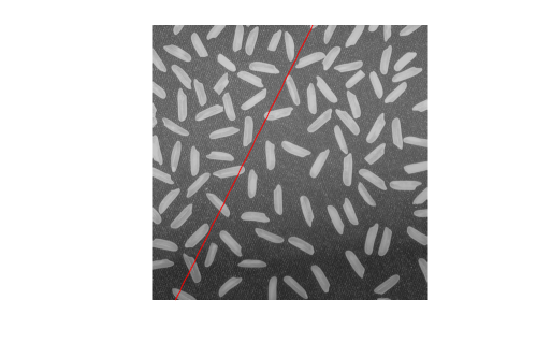# lineToBorderPoints

Intersection points of lines in image and image border

## Syntax

``points = lineToBorderPoints(lines,imageSize)``

## Description

example

````points = lineToBorderPoints(lines,imageSize)` computes the intersection points between one or more lines in an image with the image border.```

## Examples

collapse all

```I = imread('rice.png'); figure; imshow(I); hold on;```Define a line with the equation, 2 * x + y - 300 = 0.

`aLine = [2,1,-300];`

Compute the intersection points of the line and the image border.

`points = lineToBorderPoints(aLine,size(I))`
```points = 1×4 149.7500 0.5000 21.7500 256.5000 ```
`line(points([1,3]),points([2,4]));`## Input Arguments

collapse all

Line matrix, specified as an M-by-3 matrix, where each row must be in the format, [A,B,C]. This matrix corresponds to the definition of the line:

 A * x + B * y + C = 0.
M represents the number of lines.

`lines` must be `double` or `single`.

Image size, specified as a row vector in the format returned by the `size` function.

## Output Arguments

collapse all

Intersection points, returned as an M-by-4 matrix. The function returns the matrix in the format of [x1, y1, x2, y2]. In this matrix, [x1 y1] and [x2 y2] are the two intersection points. When a line in the image and the image border do not intersect, the function returns [`-1,-1,-1,-1`].

## Version History

Introduced in R2011a